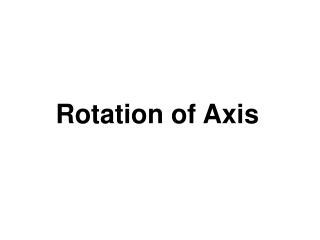DownloadDownload PresentationRotation of Axis

# Rotation of Axis

Download Presentation## Rotation of Axis

- - - - - - - - - - - - - - - - - - - - - - - - - - - E N D - - - - - - - - - - - - - - - - - - - - - - - - - - -
##### Presentation Transcript

1. Rotation of Axis

2. Identifying a Conic Section without Completing the Square • A nondegenerate conic section of the form Ax2 + Cy2 + Dx + Ey + F = 0 • in which A and C are not both zero is • a circle if A = C. • a parabola if AC = 0 • an ellipse if A = C and AC > 0, and • a hyperbola if AC < 0.

3. Solution We use A, the coefficient of x2, and C, the coefficient of y2, to identify each conic section. a. 4x2 – 25y2 – 24x + 250y – 489 = 0 A = 4 C = -25 Text Example • Identify the graph of each of the following nondegenerate conic sections. • 4x2 – 25y2 – 24x + 250y – 489 = 0 • x2 + y2 + 6x – 2y + 6 = 0 • y2 + 12x + 2y – 23 = 0 • 9x2 + 25y2 – 54x + 50y – 119 = 0 AC = 4(-25) = -100 < 0. Thus, the graph is a hyperbola.

4. Solution b. x2 + y2 + 6x – 2y + 6 = 0 Because A = C, the graph of the equation is a circle. A = 1 C = 1 c. We can write y2 + 12x + 2y – 23 = 0 as 0x2 + y2 + 12x + 2y – 23 = 0. A = 0 C = 1 AC = 0(1) = 0 Because AC = 0, the graph of the equation is a parabola. d. 9x2 + 25y2 – 54x + 50y – 119 = 0 A = 9 C = 25 AC = 9(25) = 225 > 0 Because AC > 0 and A = 0, the graph of the equation is a ellipse. Text Example cont.

5. Rotation of Axes Formulas • Suppose an xy-coordinate system and an x´y´-coordinate system have the same origin and  is the angle from the positive x-axis to the positive x´-axis. If the coordinates of point P are (x, y) in the xy-system and (x´, y´) in the rotated x´y´-system, then • x = x´cos – y´sin  • y = x´sin  + y´cos .

6. Amount of Rotation Formula • The general second-degree equation • Ax2 + Bxy + Cy2 + Dx + Ey + F = 0, B = 0 • can be rewritten as an equation in x´ and y´ without an x´y´-term by rotating the axes through angle , where

7. Writing the Equation of a Rotated Conic in Standard Form • Use the given equation Ax2 + Bxy + Cy2 + Dx + Ey + F=0, B = 0 to find cot 2. • Use the expression for cot 2 to determine , the angle of rotation. • Substitute in the rotation formulas x = x´cos – y´sin andy = x´sin  + y´cos  and simplify. • Substitute the expression for x and y from the rotation formulas in the given equation and simplify. The resulting equation should have no x´y´-term. • Write the equation involving x´ and y´ without in standard form.

8. Identifying a Conic Section without a Rotation of Axis • A nondegenerate conic section of the form Ax2 + Bxy + Cy2 + Dx + Ey + F = 0, B = 0 is • a parabola if B2 – 4AC = 0, • an ellipse or a circle if B2 – 4AC < 0, and • a hyperbola if B2 – 4AC > 0.

9. Identify the graph of 11x2 + 103 xy + y2 – 4 = 0. _ Solution We use A, B, and C, to identify the conic section. _ 11x2 + 103 xy + y2 – 4 = 0 A = 11 B = 10 3 C = 1 _ _ B2 – 4AC = (103 )2 – 4(11)(1) = 1003 – 44 = 256 > 0 Text Example Because B2 – 4AC > 0, the graph of the equation is a hyperbola.

10. Example • Write the equation xy=3 in terms of a rotated x`y`-system if the angle of rotation from the x-axis to the x`-axis is 45º Solution:

11. Example • Write the equation xy=3 in terms of a rotated x`y`-system if the angle of rotation from the x-axis to the x`-axis is 45º Solution:

12. Rotation of Axis